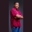Related Tags

scala
octal
communitycreator

# How to print the octal value of a number in ScalaTheodore Kelechukwu Onyejiaku

### Overview

The octal value of a number represents the value in base 10. When we print a number value to the console, we see the number in a decimal format. We can print this same number in the octal format in an easy way in Scala. All we need to do is to specify the format. This format is %o.

### Syntax

printf("%o", decimalNumber)
The syntax for printing a decimal number in octal format

### Parameters

%o: This is the format that tells the printf() function to print the number value decimalNumber in the octal format.

decimalNumber: This is the number we want to print in the octal format.

### Return value

The decimalNumber number value is returned as an octal value.

### Example

object Main extends App {
//  create some decimal numbers
var num1 = 90
var num2 = 10
var num3 = 4
var num4 = 1000

// print the decimals in octal values
printf("%d in the octal is %o", num1, num1)
printf("\n")
printf("%d in the octal is %o", num2, num2)
printf("\n")
printf("%d in the octal is %o", num3, num3)
printf("\n")
printf("%d in the octal is %o", num4, num4)
}
Print decimal numbers in octal formats

### Explanation

• Lines 3-6: We create some decimal variables and instantiate them.
• Lines 9-15: We print the decimal values in their respective octal formats using the %o format.

RELATED TAGS

scala
octal
communitycreator

CONTRIBUTORTheodore Kelechukwu Onyejiaku
RELATED COURSES

View all Courses

Keep Exploring

Learn in-demand tech skills in half the time